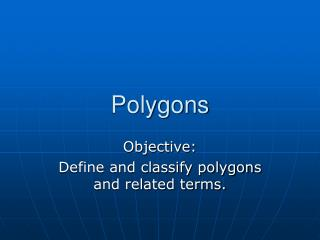DownloadDownload PresentationPolygons

# Polygons

Download Presentation## Polygons

- - - - - - - - - - - - - - - - - - - - - - - - - - - E N D - - - - - - - - - - - - - - - - - - - - - - - - - - -
##### Presentation Transcript

1. Polygons Objective: Define and classify polygons and related terms.

2. Polygons Not Polygons • Polygon • A closed figure in a plane, formed by connecting line segments end point to endpoint with each segment intersecting exactly two others. • Side: • Each line segment • Vertex: • Each endpoint, where the sides meet.

3. Classify • By number of sides • Naming a polygon: • List vertices in consecutive order. CBAED BCADE Pentagon ABCDE

4. Diagonal: • A line segment that connects two nonconsecutive vertices. • Convex • No diagonal is outside the polygon. • Concave • At least one diagonal is outside the polygon.

5. Congruent polygons • Corresponding sides and angles are congruent. (same size and shape) • Equilateral polygon • All sides have equal length. • Equiangular polygon • All angles have equal measure. • Regular polygon • Both equilateral and equiangular. Pg. 56 #1-21 Due Wednesday Quiz Tomorrow